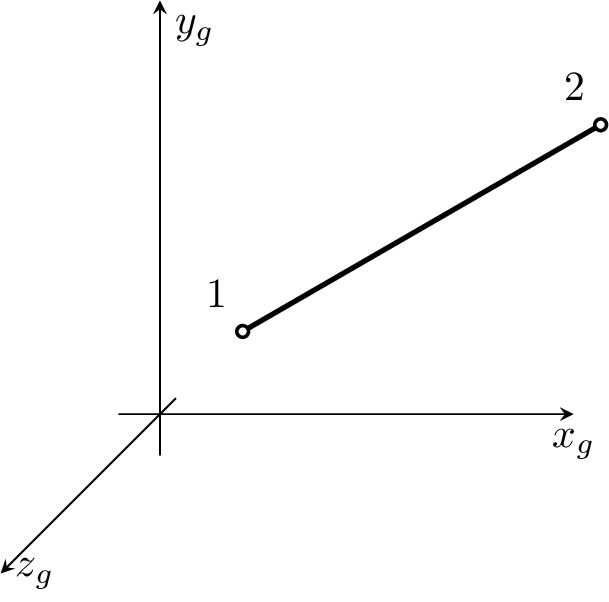#### 3.3 3D Elements

##### 3.3.1 Tetrah1ht - tetrahedral 3D element

Represents isoparametric four-node tetrahedral element. Each node has 1 degree of freedom. The same numbering convection is adopted as in mechanics, see Fig. 14. The element features are summarized in Table 52.

 Keyword Tetrah1ht Description Isoparametric, four-node tetrahedral element with linear approximation for heat transfer problems Specific parameters [NIP #(in)] Parameters NIP: allows to change the default number of integration point used. Unknowns Single dof (T_f - temperature) is required in each node. Approximation Linear approximation of temperature. Integration Integration using gauss integration formula in 1 (the default), or 4 integration points. The default number of integration point used can be overloaded using NIP parameter. Loads Body loads are supported. Boundary loads are supported and computed using numerical integration. The side and surface numbering is shown in Fig. 14. Features - CS properties - Status

Table 52: Tetrah1ht element summary

##### 3.3.2 Brick1ht - hexahedral 3D element

Represents isoparametric eight-node brick/hexahedron finite element for heat transfer problems. Each node has 1 degree of freedom. The element features are summarized in Table 53.Figure 23: Brick1ht element. Node numbers are in black, side numbers are in blue, and surface numbers are in red.

 Keyword Brick1ht Description Isoparametric, hexahedral 3D element with linear approximation for heat transfer problems Specific parameters [NIP #(in)] Parameters NIP: allows to change the default number of integration point used. Unknowns Single dof (T_f - temperature) is required in each node. Approximation Linear approximation of temperature. Integration Integration using gauss integration formula in 8 (the default), or 27 integration points. The default number of integration point used can be overloaded using NIP parameter. Loads Body loads are supported. Boundary loads are supported and computed using numerical integration. The side and surface numbering is shown in fig. (23)). Features - CS properties - Status

Table 53: Brick1ht element summary

##### 3.3.3 Brick1hmt - hexahedral 3D element

Represents isoparametric eight-node quadrilateral finite element for heat and mass (one constituent) transfer problems. Two dofs (T_f - temperature and C_1 - concentration) are required in each node. Linear approximation of temperature and mass concentration. Other features are similar to Brick1 element, see section 3.3.2.

##### 3.3.4 QBrick1ht - quadratic hexahedral 3D element

Implementation of quadratic 3d 20-node finite element. Each node has 1 degree of freedom. See section  2.8.3 for node numbering order and order of faces. The element features are summarized in Table 54.

 Keyword QBrick1ht Description Isoparametric, hexahedral 3D element with quadratic approximation for heat transfer problems Specific parameters [NIP #(in)] Parameters NIP: allows to change the default number of integration point used, possible values are 8, 27 (default) and 64. Unknowns Single dof (T_f - temperature) is required in each node. Approximation Quadratic approximation of temperature and geometry.. Integration Integration using gauss integration formula in 8, 27 (default), or 64 integration points. The default number of integration point used can be overloaded using NIP parameter. Loads - Features - CS properties - Status

Table 54: QBrick1ht element summary

##### 3.3.5 QBrick1hmt - quadratic hexahedral 3D element

The same element as QBrick1ht for heat and mass (one constituent) transfer problems. Two dofs (T_f - temperature and C_1 - concentration) are required in each node. Linear approximation of temperature and mass concentration. Other features are similar to QBrick1ht element, see section 3.3.4.